## Friday, October 6, 2017

### Another Way To Octagonize

Once in a while I have need to make a workpiece that is square in cross section into an octagon.  Last year, Greg Merritt gave us "The Octagonizer", a nifty gauge that will quickly mark out the guide lines needed to guide the material removal at the corners of square stock to make an octagon.  While I haven't made one of these gauges, I have octagonized parts using a similar method.

I read once in Dennis Laney's blog another method to octagonize square stock.  It turns out that the ratio 7:24 gives an almost exact approximation to find the location of the guide lines.

A little math first - bear with me.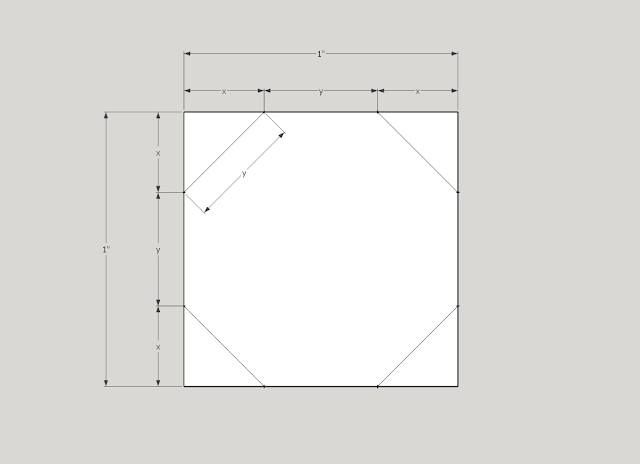A square with 1" sides and octagon lines drawn in
In the square above, each side can be divided up into three lengths; x, y and x.  "y" is the length of each of the eight sides of the octagon.  "x" is the distance from a corner of the square to a corner of the octagon.

Consider one of the small triangles formed in a corner of the square.  As Greg wrote, the Pythagorean Theorem gives us x^2 + x^2 = y^2.  We also know that for any side of this square, x + y + x = 1.

With a little algebra, we can combine these two equations and solve for x.

x = 1/[2+sqrt(2)], which is equal to 0.293.

So if you have square stock that is 1" on each side, you can mark lines 0.293" from each edge to find your octagon vertices.  For stock larger (or smaller) than 1", you can use the 0.293 as a multiplier to get the proper position.  For example, in stock that is 1 1/4" square, the lines for octagonizing will be 1.25 * 0.293, or 0.366" from the edge.

I keep an Excel sheet with decimal equivalents for every 64th of an inch.  0.366" is between 23/64" and 24/64".  So I set my marking gauge to that and mark the workpiece.Setting gauge to just over 23/64ths
Dennis Laney's method uses the fact that 7/24 (which is equal to 0.292) is almost exactly the value of "x" shown above.  This means that you can take a 24" ruler and place it diagonally on a workpiece with 0" at one edge and 24" at the other edge.24" ruler kitty corner on the stock, pencil pointing to the 7" mark
If you make a mark on the wood at the 7" mark and the 17" mark (7" from the each end of the ruler) and mark gauge lines on your stock through those points, these will be excellent guide lines for octagonizing the stock.

On some legs I'm making for a step stool, I've tapered the blanks to the top and to the bottom, with a fat part below center.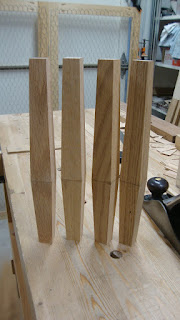Square tapered legs; 1 1/4" thick at widest part, 7/8" thick at ends
I wanted to octagonize them, so I set the marking gauge to 1 1/4" * 0.292 to mark the fattest part just over 23/64" in from the sides.  And at the ends, the marking gauge was set to 7/8" * 0.292, or just over 16/64".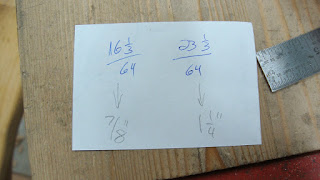Gauge mark locations for ends and middle of the tapered legMarking the middle at just over 23/64" (but not pulling the gauge along the side)And the gauge marks at the ends at just over 16/64" from the edge
Then it's a matter of connecting the gauge marks and planing to the lines.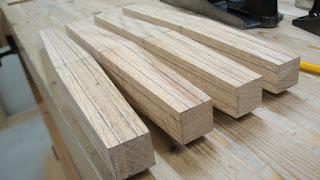Parts marked and ready for planing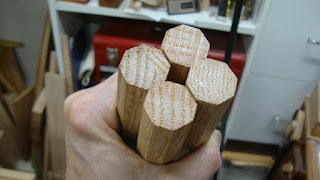Four legs tapered and octagonized
I like that I have to use the noggin while woodworking.  And (for me, anyway) it's a bonus when I get to use some algebra and geometry in the process.

1.1.